# Test: Digital Circuits & Flip Flops

## 15 Questions MCQ Test GATE Computer Science Engineering(CSE) 2023 Mock Test Series | Test: Digital Circuits & Flip Flops

Description
Attempt Test: Digital Circuits & Flip Flops | 15 questions in 45 minutes | Mock test for Computer Science Engineering (CSE) preparation | Free important questions MCQ to study GATE Computer Science Engineering(CSE) 2023 Mock Test Series for Computer Science Engineering (CSE) Exam | Download free PDF with solutions
*Answer can only contain numeric values
QUESTION: 1

### A logic network has two data inputs A and B, and two control inputs C0 and C1. It implements the function F according to the following table.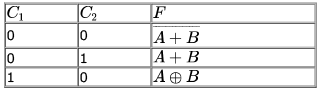Implement the circuit using one 4 to 1 Multiplexer, one 2-input Exclusive OR gate, one 2-input AND gate, one 2-input OR gate and one Inverter.

Solution: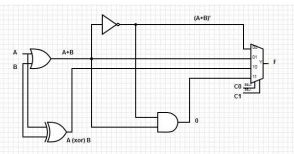This is the implementation asked in question
C0 = 0 , C1 = 0  line 00 will be selected and F will give (A+B)'
C0 = 0 , C1 = 1  line 01 will be selected and F will give (A+B)
C0 = 1 , C1 = 0  line 10 will be selected and F will give (A⊕B)
C0 = 1 , C1 = 1 line 11 will be selected and F will give (A+B)'.(A+B) =0

QUESTION: 2

### In the following truth table, V = 1 if and only if the input is valid.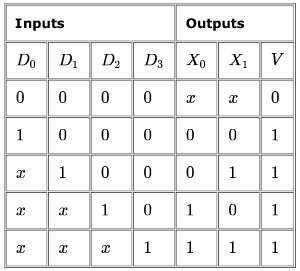What function does the truth table represent?

Solution:

For 2n inputs we are having n outputs.
Here n = 2.

QUESTION: 3

### Consider the following combinational function block involving four Boolean variables x,y,a,b where x,a,b are inputs and y is the output. if(x, a,b,y) {    if( x is 1) y=a;     else y=b; } Which one of the following digital logic blocks is the most suitable for implementing this function?

Solution:

2X1 multiplexer

QUESTION: 4

How many pulses are needed to change the contents of a 8-bit up counter from 10101100 to 00100111 (rightmost bit is the LSB)?

Solution:

As in a 28 Counter the range would be from 0-255. Hence to go from 10101100 (172) to 00100111 (39) , the counter has to go initially from 172 to 255 and then from 0 to 39.
Hence to go from 172 to 255,  255-172 = 83 Clock pulses would be required. then from 255 to 0 , again 1 clock pulse would be required.Then from 0 to 39 , 39 clock pulses would be required. Hence in total 83+1+39 =123 Clock pulses would be required.

QUESTION: 5

The minimum number of D flip-flops needed to design a mod-258 counter is

Solution:

mod 258 counter has 258 states. We need to find no. of bits to represent 257 at max.
2n > 258 ⇒ n > 9.

QUESTION: 6

Let = k = 2n. A circuit is built by giving the output of an n-bit binary counter as input to an n- to-2n bit decoder. This circuit is equivalent to a

Solution:

In binary counter of n bits can count upto 2n numbers..when this op from counter  is fed to decoder one of n out of 2n will be activated..so this arrangement of counter and decoder is behaving as 2n(k) ring counter..

QUESTION: 7

Consider a 4-bit Johnson counter with an initial value of 0000. The counting sequence of this counter is

Solution:

0000 - 0
1000 - 8
1100 - 12
and so on.

QUESTION: 8

The next state table of a 2-bit saturating up-counter is given below.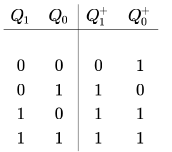The counter is built as a synchronous sequential circuit using T flip-flops. The expressions for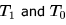are

Solution: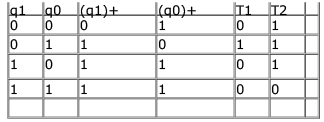By using above excitation table, T1=q1'q0,
T2=(q1q0)'=q1'+q0'

QUESTION: 9

The dual of a Boolean function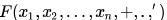written as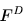is the same expression as that of F with + and swapped. F is said to be self-dual if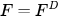. The number of self-dual functions with  Boolean variables is

Solution:

A function is self dual if it is equal to its dual (A dual function is obtained by interchanging.  and +).
For self-dual functions,
1. Number of min terms equals number of max terms
2. Function should not contain two complementary minterms  - whose sum equals 2n - 1, where n is the number of variables.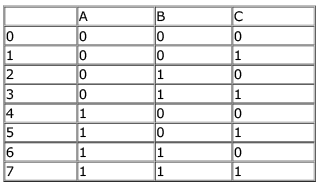so here (0,7) (1,6) (2,5) (3,4) are complementary terms so in self-dual we can select any one of them but not both.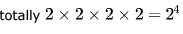possibility because say from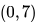we can pick anyone in minterm but not both.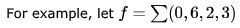NOTE:here i have taken only one of the complementary term for min term from the sets.
so remaining numbers will go to MAXTERMS
For above example, 24 = 16 self dual functions are possible
so if we have N variables, total Minterms possible is 2n
then half of them we selected so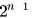.
and now we have 2 choices for every pair for being selected.
so total such choices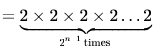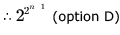QUESTION: 10

Latches constructed with NOR and NAND gates tend to remain in the latched condition due to which configuration feature?

Solution:

Both inputs of a latch are directly connected to the other’s output. Such types of structure is called cross coupling and due to which latches remain in the latched condition.

*Answer can only contain numeric values
QUESTION: 11

Find the maximum clock frequency at which the counter in the figure below can be operated. Assume that the propagation delay through each flip flop and each AND gate is 10 ns. Also assume that the setup time for the J K inputs of the flip flops is negligible.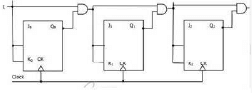Solution:

In a JK flip flop the output toggles when both J and K inputs are 1. So, we must ensure that with each clock the output from the previous stage reaches the current stage. From the figure, there is an AND gate between each stage and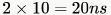(10ns for output to reach the gate and 10ns for the output of AND gate to reach the next flipflop) isneeded for the output to reach the next stage. So, minimum time period needed for clock is 20ns which would mean a maximum clock frequency of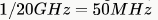QUESTION: 12

The exponent of a floating-point number is represented in excess-N code so that:

Solution:

Ans : C) The smallest number is represented by all zeros.
In computer system, a floating-point number is represented as S E M, i.e. using Sign bit, Exponent bits and Mantissa bits.
The exponent can be a positive as well as a negative number. So to represent negative number we can use 1's complement or 2's complement. Better choice would be 2's complement.
If we use 2's complement system to represent exponent, then problem will arise while comparing 2 floating point numbers.
For example, if exponent of the 2 numbers are negative then for comparing we will have to convert them into positive number.
So to avoid this extra work, excess-N code is used so that all exponent can be represented in positive numbers, starting with 0.

QUESTION: 13

The following is a scheme for floating point number representation using 16 bits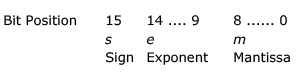Let s, e, and m be the numbers represented in binary in the sign, exponent, and mantissa fields respectively. Then the floating point number represented is:What is the maximum difference between two successive real numbers representable in this system?

Solution:

Maximum difference between two successive real number will occur at extremes. This is because numbers are represented upto mantissa bits and as the exponent grows larger, the difference gets multiplied by a larger value. (The minimum difference happens for the least positive exponent value).
Biasing will be done by adding 31 as given in question. So, actual value of exponent will be represented value - 31. Also, we can not have exponent field as all 1's as given in question (usually taken for representing infinity, NAN etc). So, largest value that can be stored is 111110 = 62.

Largest number will be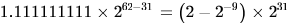Second largest number will be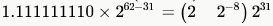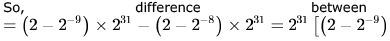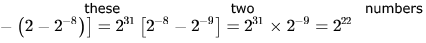QUESTION: 14

Consider the following floating-point format.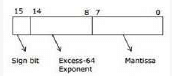Mantissa is a pure fraction in sign-magnitude form.

The decimal number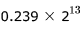has the following hexadecimal representation (without normalization and rounding off):

Solution:

answer = option D in both questions.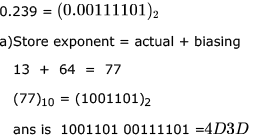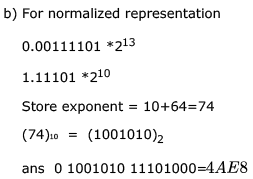QUESTION: 15

Consider the following floating-point format.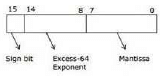Mantissa is a pure fraction in sign-magnitude form.
The normalized representation for the above format is specified as follows. The mantissa has an implicit 1 preceding the binary (radix) point. Assume that only 0’s are padded in while shifting a field.

The normalized representation of the above number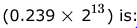Solution:

For finding normalised representation we need to find unnormalised one first..So we have , 0.239 * 213 as the number ..So we find the binary equivalent of 0.239 till 8 digits as capacity of mantissa field is 8 bits..
So following the procedure we have , 0.239 * 2 = 0.478
0.478 * 2 = 0.956
0.956 * 2 = 1.912
0.912 * 2 = 1.824
0.824 * 2 = 1.648
0.648 * 2 = 1.296
0.296 * 2 = 0.512
0.512 * 2 = 1.024
We stop here as we have performed 8 iterations and hence 8 digits of mantissa of unnormalised number is obtained.So we have

Mantissa of given number  =  0011 1101
So the number can be written as : 0.00111101 * 213 Now we need to align the mantissa towards left to get normalised number..And in the question it is mentioned that during alignment process 0's will be padded in the right side as a result of mantissa alignment to left..
So to get normalised number , we align to left 3 times , so new mantissa = 11101 000
So exponent will also decrease by 3 hence new exponent  =  10
So normalised number = 1.11101000 * 210
So actual exponent = 10
Given excess 64 is used..So bias value = 64

So exponent field value = 74
And of course sign bit = 0 being a positive number.
Thus the final representation of number  =  0 1001010 11101000         =  0100 1010 1110 1000
=  (4AE816)
Hence D) should be the correct answer.Use Code STAYHOME200 and get INR 200 additional OFF Use Coupon Code